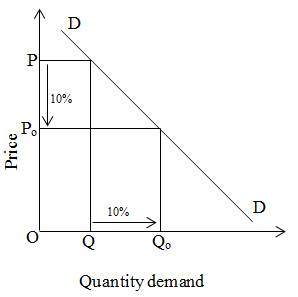# Unitary elastic demand

If percentage change in price commodity is equal to percentage change in quantity demand then it is known as unitary elastic demand. We can explain it by the given figure.In the above figure, when price is OP then demand is OQ. When the price decreases by 10% price becomes Po. At that time quantity demand increases by 10% and demand becomes Qo.